# Vlookup for Duplicate Values and Return the Matches in Excel [8 Cases]

To find the duplicate values in Excel, we need to Vlookup (Vertical Lookup) through columns. Sometimes we need just to identify the duplicate values. But sometimes we need to pull the duplicates out of the data table too. Different scenarios can arise regarding looking up duplicate values in Excel. In this article, I have discussed 8 relevant cases to vlookup for duplicate values and return the matches in Excel.

### Introduction to the Dataset

In the following picture, the Product List 1 and Product List 2 columns contain different product names. There are some common product names between the two columns.

My target is to show you how can you find these matched product names.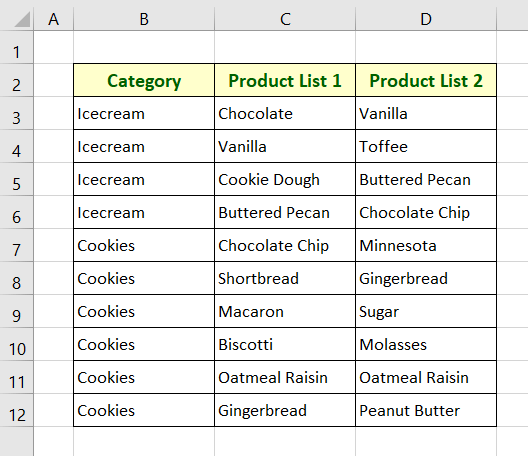## Case #1: Vlookup for Duplicate Values to Pull Data Based on Matches Using VLOOKUP & IFERROR Functions in Excel

In this part, I will vlookup between the columns Product List 1 and Product List 2. Then I will extract the product names from the Product List 2 column that matches with the Product List 1 column contents.

For this purpose, I will use a formula using the VLOOKUP and IFERROR functions.

Syntax

`=IFERROR(VLOOKUP(lookup_value, table_array, col_index_num, [range_lookup])," ")`

Usage Guide

Step_1: Create a separate column to extract the duplicate values.

Here, I’ve created a column named “Duplicate Products”.

Step_2: Copy the following formula of IFERROR and VLOOKUP functions in the top cell of the new column.

`=IFERROR(VLOOKUP(D3,\$C\$3:\$C\$12,1,FALSE)," ")`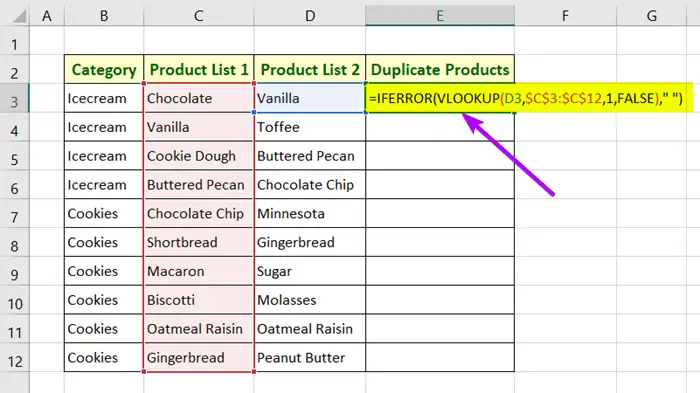Formula Breakdown

• D3 is the lookup value which is the top cell of the column Product List 2.
• \$C\$3:\$C\$12 is the table array which is the range of the column Product List 1.
• 1 is the column index number which refers to the first column in the range \$C\$3:\$C\$12.
• FALSE is responsible for finding the exact matches between Product List 1 and Product List 2.
• Here, the VLOOKUP function is used to vlookup and find duplicates. If the function leaves any error that replaces the IFERROR function with blanks (“”).

Step_3: Press ENTER to insert the formula.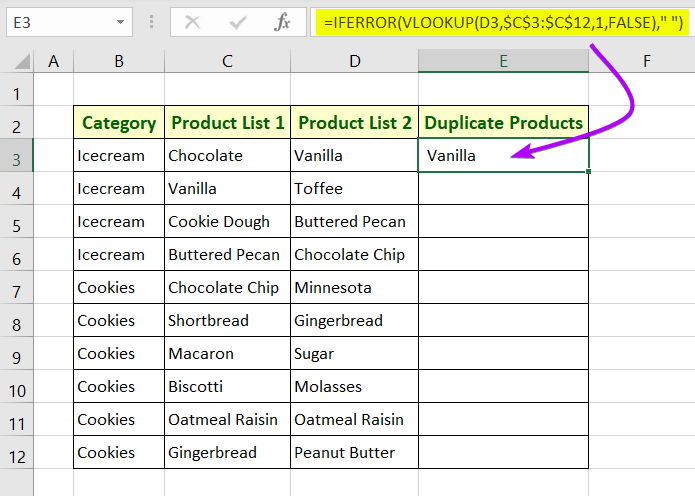Step_4: Keep the mouse cursor at the right-bottom corner of the cell where you have just inserted the formula.

A plus-like icon (+)  will appear.

Step_5: To copy the formula down the entire column double-click on the icon.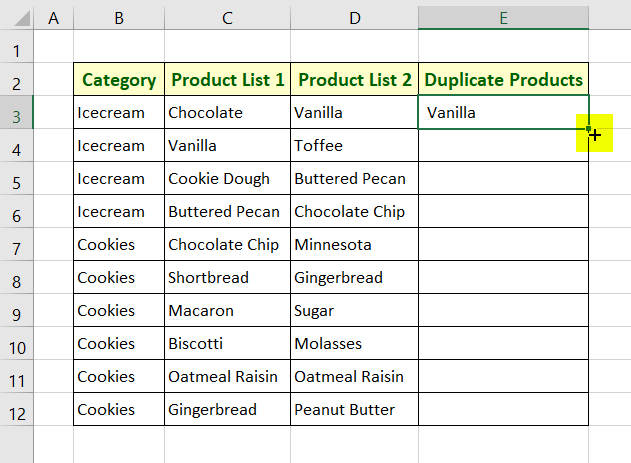Final Result

Duplicate values from the Product List 2 column are extracted to the column “Duplicate Products”.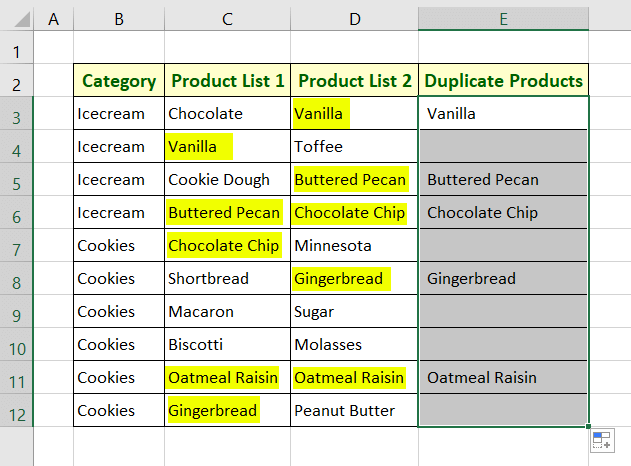🔗 How to Find Duplicates in Two Columns in Excel (7 Methods)

## Case #2: Vlookup for Duplicate Values in Excel and Return Row Numbers of the Matched Data

Here, I will compare the column Product List 2 with Product List 1. Then I will return the row number of the value from Product List 1 that matches with a value from Product List 2.

I will use a formula of IFERROR, CONCATENATE, SUM, & MATCH functions to perform the operation.

Syntax

`=IFERROR(CONCATENATE("Matched with Row ",SUM(MATCH(lookup_value, lookup_array, [match_type]),adjust_num)),"")`

Usage Guide

Step_1: Create a new column to keep the row numbers.

Step_2:  Write the formula below at the top cell of the new column.

`=IFERROR(CONCATENATE("Matched with Row ",SUM(MATCH(D3,\$C\$3:\$C\$12,FALSE),2)),"")`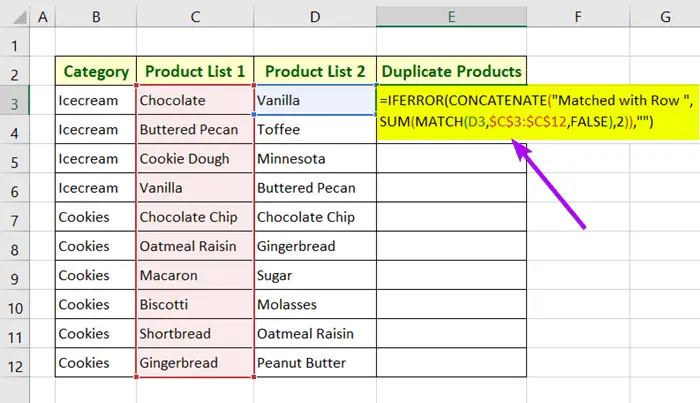Formula Breakdown

• D3 is the lookup value which is the top cell of the column Product List 2.
• \$C\$3:\$C\$12 is the table array which is the range of the column Product List 1.
• FALSE is responsible for finding the exact matches between Product List 1 and Product List 2.
• 2 is the adjustment_number. You need to change this number according to your need.
• The SUM function adds 2 with the output of the MATCH function. Thus, we get the correct row number.
• The CONCATENATE function merges the phrase “Matched with Row “ with the output of SUM(MATCH(D3,\$C\$3:\$C\$12,FALSE),2).
• Finally, the IFERROR function replaces any error left by the MATCH function with blanks (“”).

Step_3: Hit ENTER.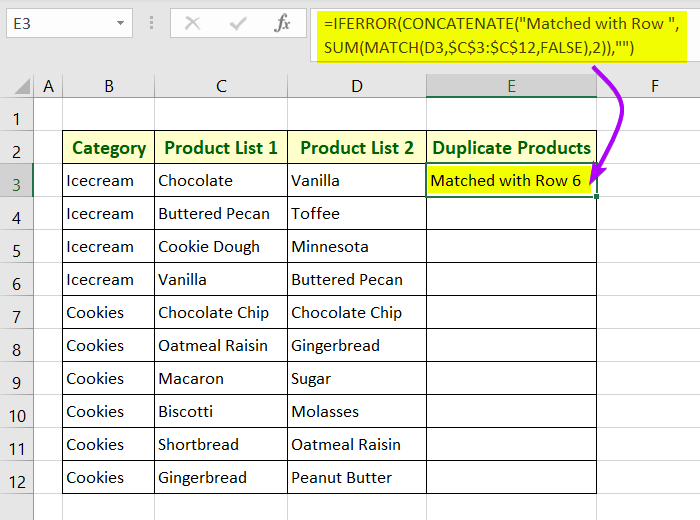Step_4: Copy down the above formula to the entire column. For this, you can double-click on the Fill Handle icon or simply drag it down.

Final Result

In the column named “Duplicate Product”, you will see the row number of the data from the Product List 1 column that matches with the data from the column “Product List 2”.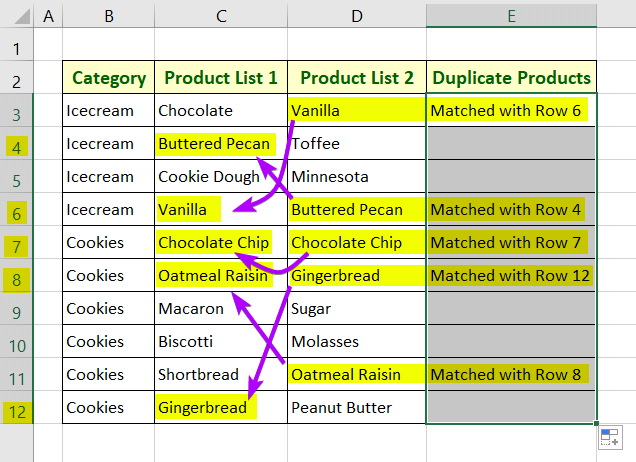🔗 How to Compare Rows in Excel for Duplicates (7 Ways)

## Case #3: Vlookup for Duplicate Values to Return Data Based on Matches Using MATCH, IF, & IFERROR Functions in Excel

In this case, I will write a formula using the MATCH, IF, & IFERROR functions. This formula will look for the duplicate values between Product List 1 and Product List 2. Then it will return the duplicate product names from Product List 2 into the column “Duplicate Products”.

Syntax

`=IFERROR(IF(MATCH(lookup_value, lookup_array, [match_type])>0,D3,""),"")`

Usage Guide

Step_1: Write down the following formula in the top cell of the column, “Duplicate Products”.

`=IFERROR(IF(MATCH(D3,\$C\$3:\$C\$12,FALSE)>0,D3,""),"")`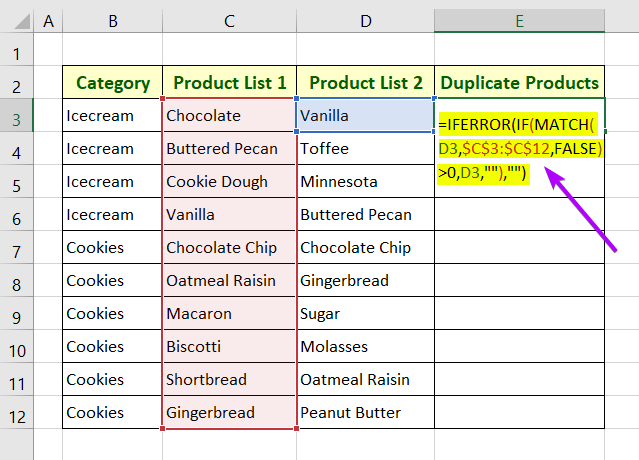Formula Breakdown

• D3 is the lookup value which is the top cell of the column Product List 2.
• \$C\$3:\$C\$12 is the table array which is the range of the column Product List 1.
• FALSE is responsible for finding the exact matches between Product List 1 and Product List 2.
• IF(MATCH(D3,\$C\$3:\$C\$12,FALSE)>0,D3,””) returns the value in cell D3 if MATCH(D3,\$C\$3:\$C\$12,FALSE)>0 becomes true. Otherwise, it returns blanks (“”).
• Finally, the IFERROR function replaces any error left by the MATCH function with blanks (“”).

Step_2: Press the ENTER button to insert the formula in cell E3.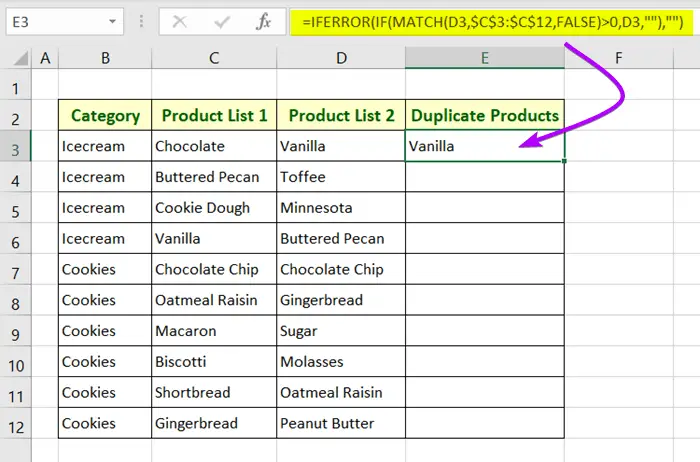Step_3: Copy down the above formula to the entire column. For this, you can double-click on the Fill Handle icon or simply drag it down.

Final Result

Duplicate values from the Product List 2 column are returned to the column “Duplicate Products”.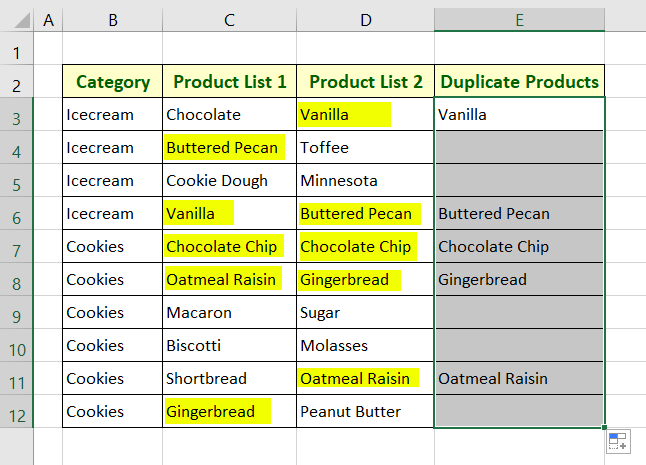🔗 How to Find Similar Text in Two Columns in Excel (5 Ways)

## Case #4: Vlookup in Two Columns and Identify Duplicate & Unique Values in Excel

In this case, I will write a formula to Vlookup between Product List 1 and 2. Then in the column, “Duplicate Products” I will report either Duplicate or Unique against the data of Product List 2.

Syntax

`=IF(ISNA(VLOOKUP(lookup_value, table_array, col_index_num, [range_lookup])),Value_If_True,Value_If_False)`

Usage Guide

Step_1: Copy the formula below in the top cell of the column, “Duplicate Products”.

`=IF(ISNA(VLOOKUP(D3,\$C\$3:\$C\$12,1,FALSE)),"Unique","Duplicate")`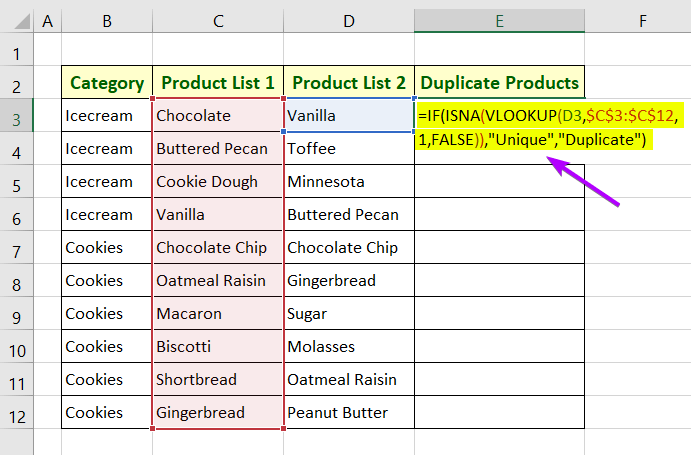Formula Breakdown

• D3 is the lookup value which is the top cell of the column Product List 2.
• \$C\$3:\$C\$12 is the table array which is the range of the column Product List 1.
• 1 is the column index number which refers to the first column in the range \$C\$3:\$C\$12.
• FALSE is responsible for finding the exact matches between Product List 1 and Product List 2.
• The ISNA function returns TRUE if VLOOKUP(D3,\$C\$3:\$C\$12,1,FALSE) returns any #N/A error. Otherwise, If returns FALSE.
• The IF function returns Unique if the ISNA function returns TRUE. Otherwise, it returns Duplicate.

Step_2: Press ENTER.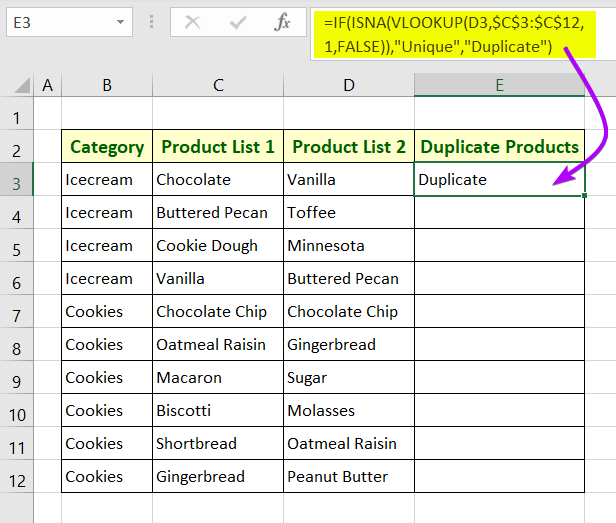Step_3: Copy down the above formula to the entire column. For this, you can double-click on the Fill Handle icon or simply drag it down.

Final Result

In the “Duplicate Products” column, the formula returns Duplicate if any value of Product List 2 matches with Product List 1. Otherwise, It returns Unique.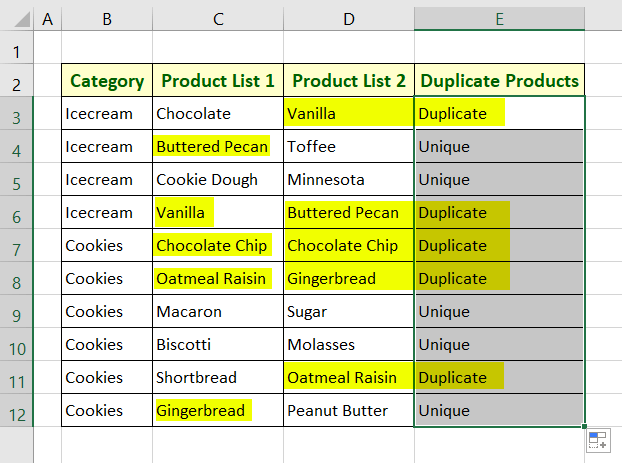🔗 5 Ways to Find Matching Values in Two Worksheets in Excel

## Case #5: Create a Search Box Using VLOOKUP, IF, & IFNA Functions to Vlookup for Duplicate Values in Excel

Now I will show you to create a search box where you can insert product names. Then a formula will determine whether that product name is a duplicate or not.

Syntax

`=IF(IF(ISNA(VLOOKUP(lookup_value, table_array, col_index_num, [range_lookup]))),0,1)+IF(ISNA(VLOOKUP(lookup_value,table_array, col_index_num, [range_lookup]))),0,1)=2,Value_If_True,Value_If_False)`

Usage Guide

Step_1: Type the formula below in cell D15.

`=IF(IF(ISNA(VLOOKUP(D14,C3:C12,1,FALSE)),0,1)+IF(ISNA(VLOOKUP(D14,D3:D12,1,FALSE)),0,1)=2,"Duplicate","Unique")`

Step_2: Hit ENTER.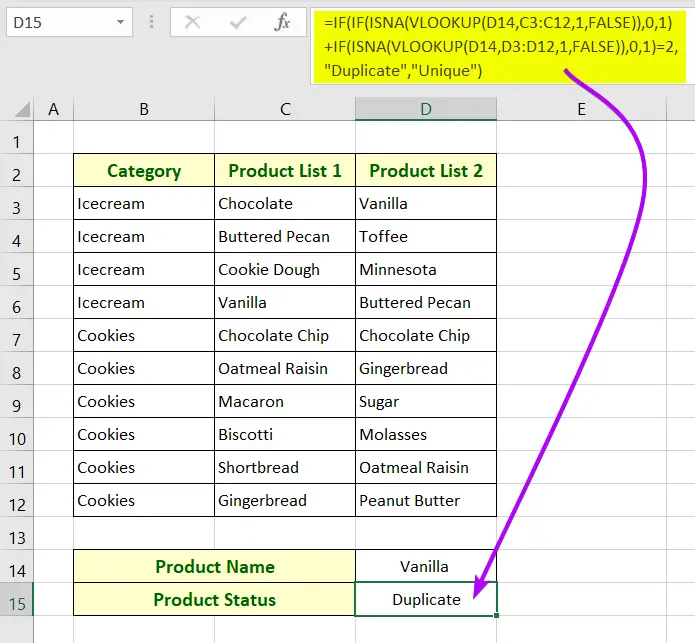Formula Breakdown

• D14 is the lookup value that refers to a product name to justify whether it’s unique or not.
• C3:C12 is the table array which is the range of the column Product List 1.
• D3:D12 is the table array which is the range of the column Product List 2.
• 1 is the column index number which refers to the first column for both ranges C3:C12 & D3:D12.
• FALSE is responsible for finding the exact matches between Product List 1 and Product List 2.
• The ISNA function returns TRUE if the VLOOKUP function returns any #N/A error. Otherwise, If returns FALSE.
• The IF function returns 0 if the ISNA function returns TRUE. Otherwise, it returns 1.
• IF(ISNA(VLOOKUP(D14,C3:C12,1,FALSE)),0,1) returns 0 if the value in cell D14 matches with any data in the range C3:C12.
• IF(ISNA(VLOOKUP(D14,D3:D12,1,FALSE)),0,1) returns 0 if the value in cell D14 matches with any data in the range D3:D12.
• If the value in cell D14 exists both in Product List 1 and Product List 2, then IF(ISNA(VLOOKUP(D14,C3:C12,1,FALSE)),0,1)+IF(ISNA(VLOOKUP(D14,D3:D12,1,FALSE)),0,1) will return 2. Otherwise, it will return either 0 or 1.
• If IF(ISNA(VLOOKUP(D14,C3:C12,1,FALSE)),0,1)+IF(ISNA(VLOOKUP(D14,D3:D12,1,FALSE)),0,1)=2 becomes true the first IF function will return Duplicate. Otherwise, it will return Unique.

🔗 Find, Highlight, and Remove Duplicates in Excel [Step-by-Step]

## Case #6: Vlookup  for Duplicate Values Between Two Worksheets and Identify Duplicate & Unique Data in Excel

In the worksheet named “Formula 6A”, there are two columns named “Category” and “Product List 1”.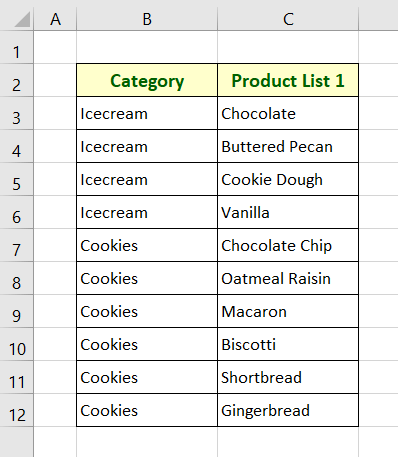In another worksheet named “Formula 6B”, there are two columns named “Category” and “Product List 2”.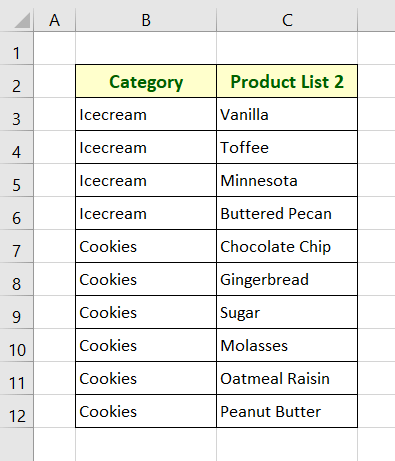Now I will show you how you can Vlookup between these two datasets from two different worksheets.

I have created an additional column named “Product Status” adjacent to the column, “Product List 1” in the worksheet “Formula 6A”.

In this column, I will return “Duplicate” if any value from Product List 1 matches that of Product List 2.

For this purpose, I will write a formula using the VLOOKUP, ISERROR, and IF functions.

Syntax

`=IF(ISERROR(VLOOKUP(lookup_value, table_array, col_index_num, [range_lookup])),Value_If_True,Value_If_False)`

Usage Guide

Step_1: Copy the following formula in the top cell of the column, “Product Status”.

`=IF(ISERROR(VLOOKUP(C3,'Formula 6B'!\$C\$3:\$C\$12,1,0)),"Unique", "Duplicate")`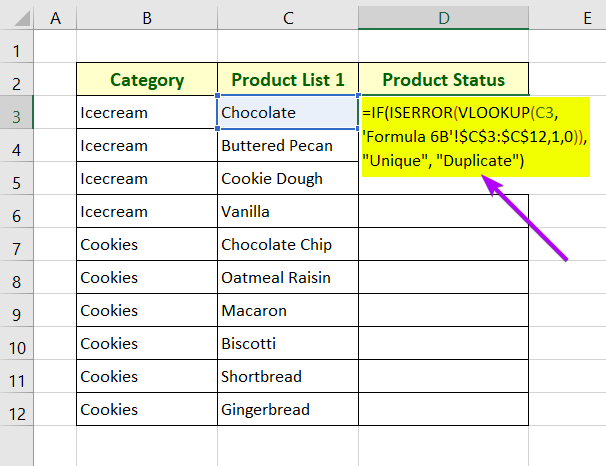Formula Breakdown

• C3 is the lookup value which is the top cell of the column Product List 1.
• ‘Formula 6B’ is the other worksheet name.
• \$C\$3:\$C\$12 is the table array which is the range of the column Product List 2.
• 1 is the column index number which refers to the first column in the range \$C\$3:\$C\$12.
• 0 is responsible for finding the exact matches between Product List 1 and Product List 2.
• The IF function return “Duplicate” if any value from Product List 1 matches that of Product List 2 from the worksheet “Formula 6B”. Otherwise, it returns “Unique”.

Step_2: Press ENTER to insert the formula in cell D3.

It will return “Unique” there.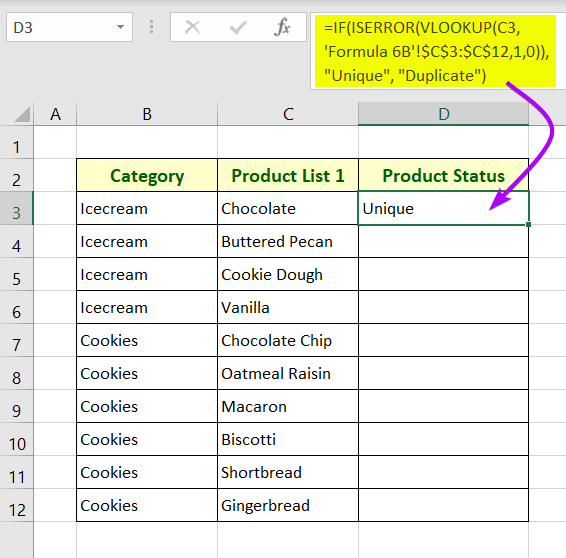Step_3: Double-click on the Fill Handle icon to copy the above formula down the entire column, “Product Status”.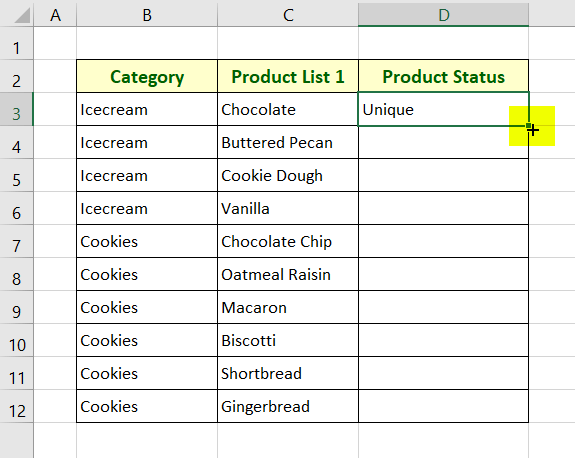Final Result

In the column, “Product Status”, the formula will return “Unique” if any value from Product List 1 matches that of Product List 2. Otherwise, it returns “Duplicate”.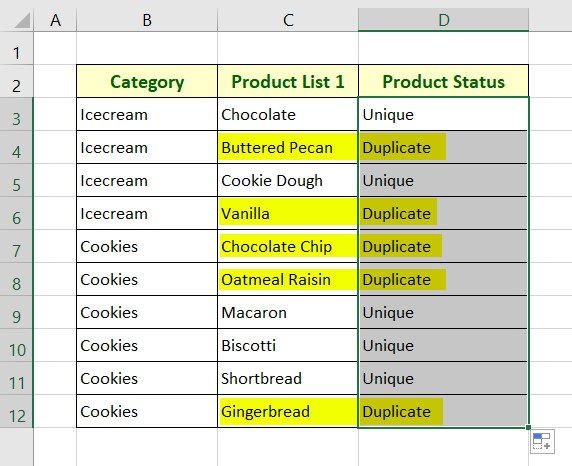🔗 How to Remove Duplicates in Excel [13 + Different Methods]

## Case #7: Vlookup  for Duplicate Values Between Two Workbooks and Identify Duplicate & Unique Data in Excel

The dataset in the picture below is from a workbook named “Book2.xlsm”.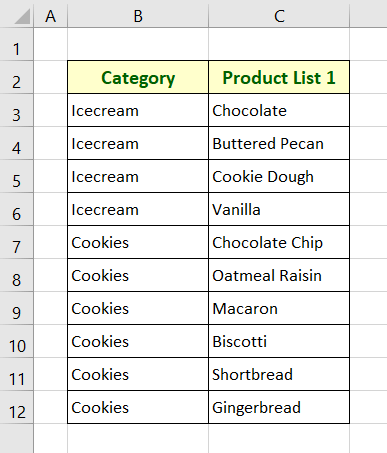And the second dataset in the following picture is from a workbook named “Book1.xlsx”.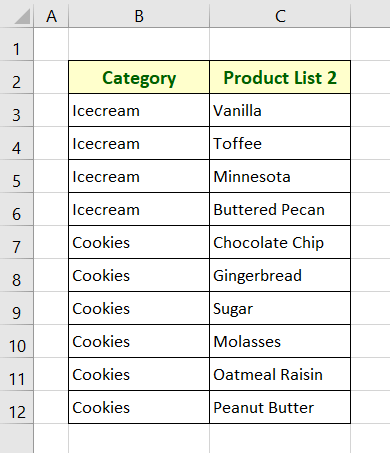Now I will show you to vlookup between these two datasets from two different workbooks.

I have created an extra column named “Product Status” right next to Product List 1 in the workbook “Book2.xlsm”.

To perform the task, I have created a formula using the VLOOKUP, ISERROR, & IF functions.

Syntax

`=IF(ISERROR(VLOOKUP(lookup_value, table_array, col_index_num, [range_lookup])),Value_If_True,Value_If_False)`

Usage Guide

Step_1: Type the following formula in cell D3 of the Product Status column.

`=IF(ISERROR(VLOOKUP(C3,'[Book1.xlsx]Formula 7B'!\$C\$3:\$C\$12,1,0)),"Unique", "Duplicate")`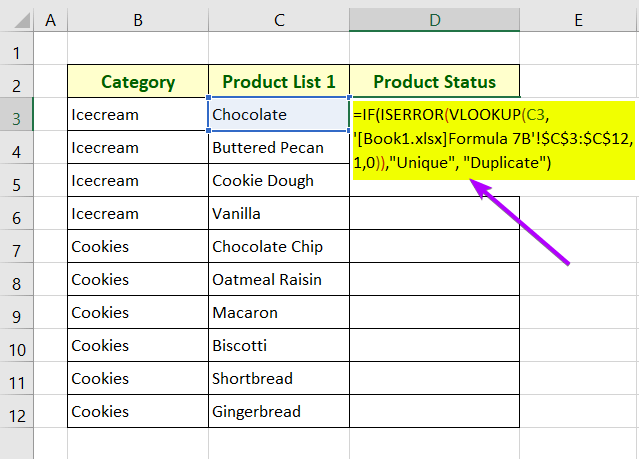Formula Breakdown

• C3 is the lookup value which is the top cell of the column Product List 1.
• [Book1.xlsx] is the other workbook name.
• ‘Formula 7B’ is the worksheet name of the workbook “Book1.xlsx”.
• \$C\$3:\$C\$12 is the table array which is the range of the column Product List 2.
• 1 is the column index number which refers to the first column in the range \$C\$3:\$C\$12.
• 0 is responsible for finding the exact matches between Product List 1 and Product List 2.
• The IF function return “Duplicate” if any value from the Product List 1 matches that of Product List 2 from the worksheet “Formula 7B” of the workbook “Book1.xlsx”. Otherwise, it returns “Unique”.

Step_2: Hit ENTER.

It will return “Unique” in cell D3.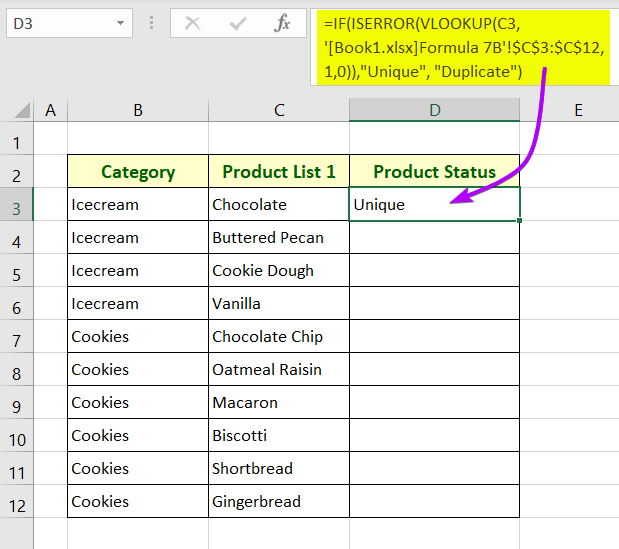Step_3: Double-click on the Fill Handle icon to AutoFil the above formula to the entire column.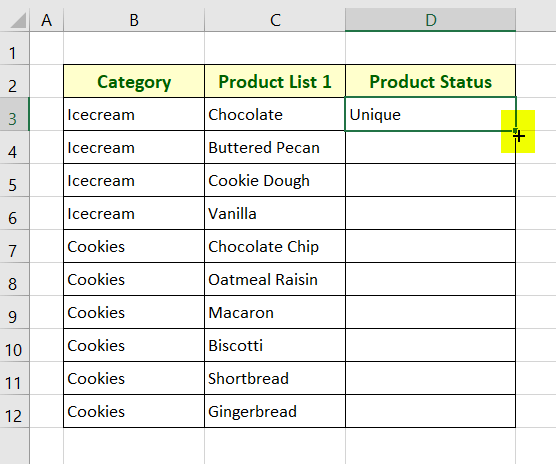Final Result

In the column, “Product Status”, the formula will return “Unique” if any value from Product List 1 matches that of Product List 2. Otherwise, it returns “Duplicate”.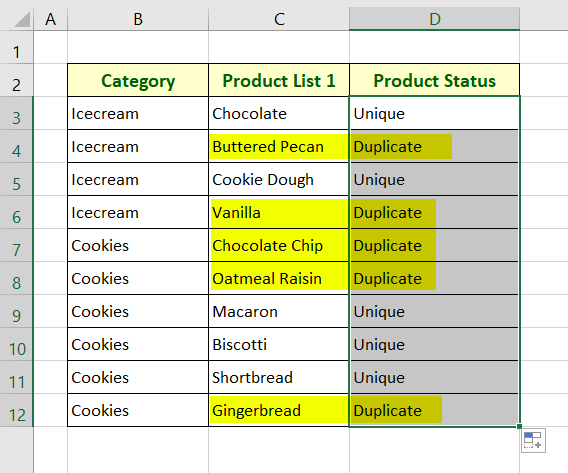🔗 4+ Ways to Find Duplicates in a Column and Delete Rows in Excel

## Case #8: Create a User-Defined Function Using VBA Script to Vlookup for Duplicate Values and Return 1st, 2nd, 3rd, etc. Occurrences in Excel

Now, I will create a user-defined function using a VBA script. The user-defined function will vlookup for duplicate values between Product List 1 and Product List 2.

What’s special about this user-defined function is that it can identify 1st, the 2nd, and 3rd occurrences of a duplicate value. Based on the identification, it can return those values in a separate column.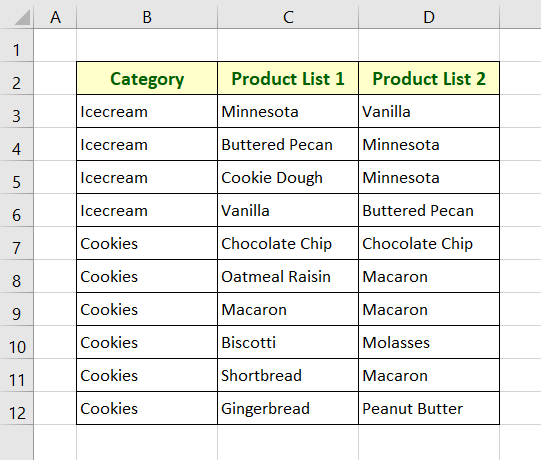Usage Guide

Step_1: Open the Visual Basic Editor.

You can open it using one of the 3 ways,

• Right-click on the sheet name and choose View Code.
• Press ALT + F11.
• Click on Visual Basic in the Code group of the Developer tab from the main ribbon.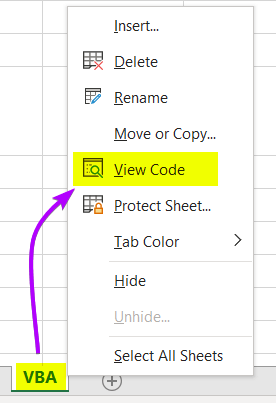Step_2: Open a new module. To do that, click on the Insert tab and choose Module.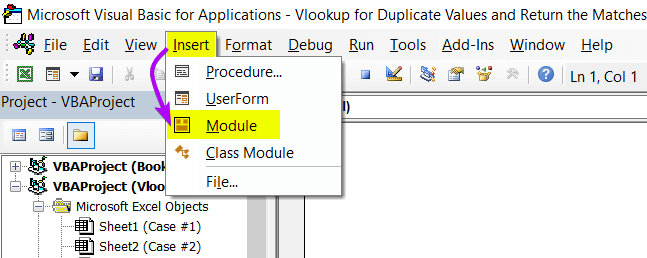Step_3: Copy the VBA script below.

``````Function NthVlookup(x1, x2 As Range, cx3 As Integer, x4)

Dim xRow1 As Long
Dim xVal1 As Integer
Dim xFound1 As Boolean

NthVlookup = " "

With x2
For xRow1 = 1 To .Rows.Count
If .Cells(xRow1, 1).Value = x1 Then
xVal1 = xVal1 + 1
End If
If xVal1 = x4 Then
NthVlookup = .Cells(xRow1, cx3).Text
Exit Function
End If
Next xRow1
End With

End Function``````

Step_4: Press CTRL + V to paste the code into the Visual Basic Editor.

Step_5: Save the workbook as Excel Macro-Enabled Workbook from the File tab.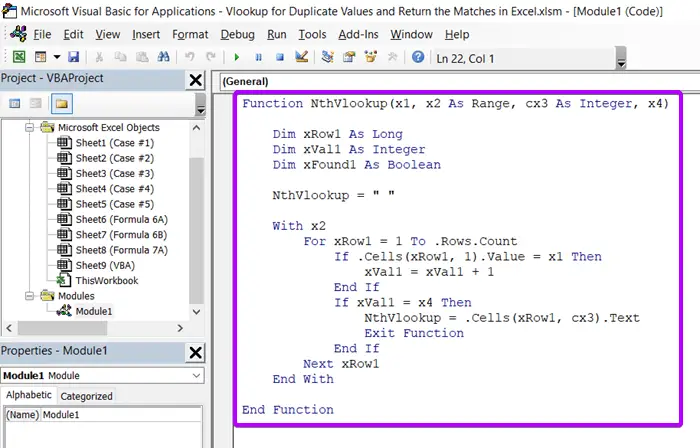Now come back to the worksheet where you want to vlookup.

### A. Return the Duplicates for the 1st Occurrence

Use the following formula to return duplicates from Product List 1 having only one copy exists in Product List 2.

Syntax

`=NthVlookup(lookup_value, table_array, col_index_num,occurrence)`

Usage Guide

Step_1: Insert the following formula in the top cell of column “1st Occurrence”.

`=NthVlookup(C3,\$D\$3:\$D\$12,1,1)`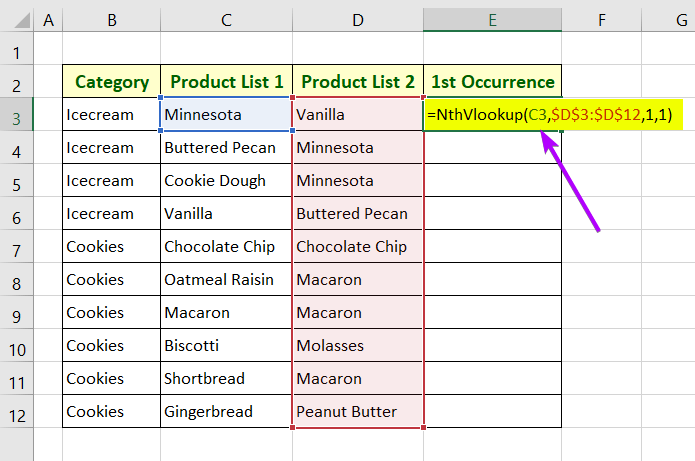Formula Breakdown

• C3 is the lookup value which is the top cell of the column Product List 1.
• \$D\$3:\$D\$12 is the table array which is the range of the column Product List 2.
• 1 is the column index number which refers to the first column in the range \$D\$3:\$D\$12.
• 1 is the occurrence number that refers to the 1st occurrence.

Step_2: Press ENTER.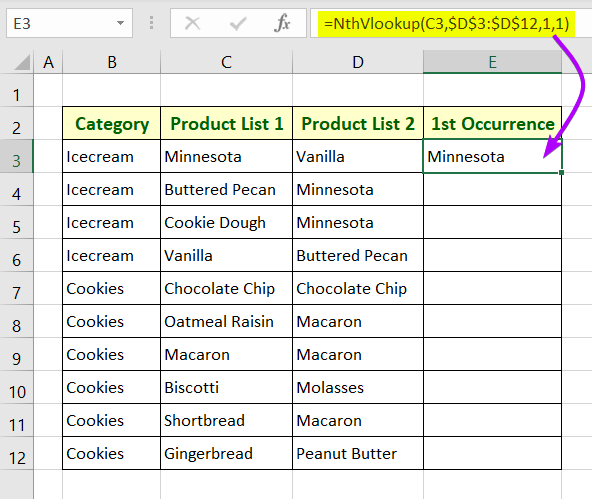Step_3: Double-click on the Fill Handle icon to copy the formula down the entire column.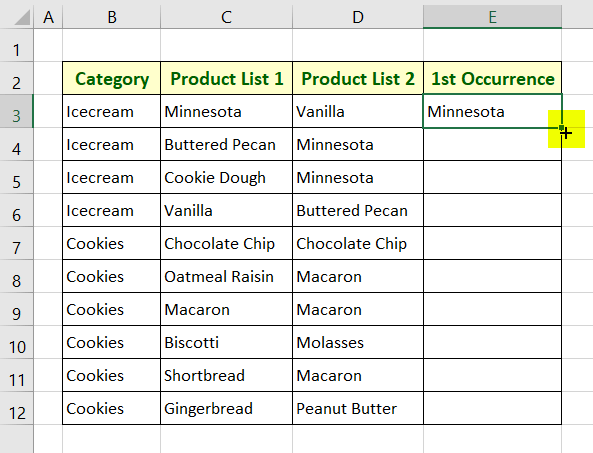Final Result

In the 1st Occurrence column, you will see only the duplicates from Product List 1 having only one copy exists in Product List 2.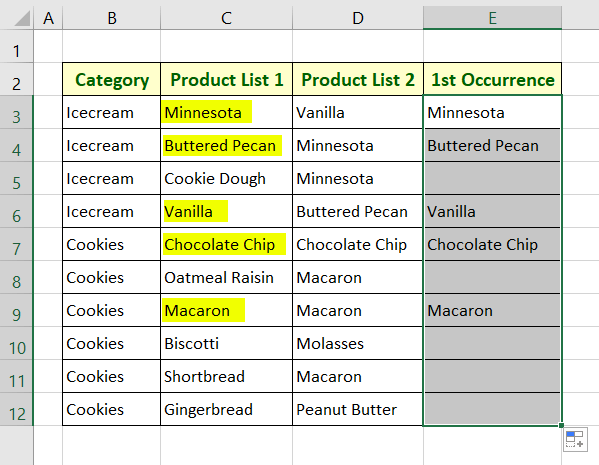### B. Return the Duplicates for the 2nd Occurrence

Use the following formula to return duplicates from Product List 1 having at least two copies exist in Product List 2.

Syntax

`=NthVlookup(lookup_value, table_array, col_index_num,occurrence)`

Usage Guide

Step_1: Insert the following formula in the top cell of column “2nd Occurrence”.

`=NthVlookup(C3,\$D\$3:\$D\$12,1,2)`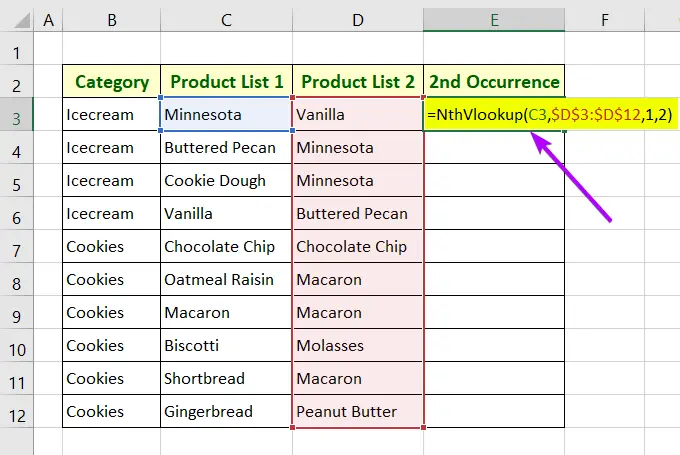Formula Breakdown

• C3 is the lookup value which is the top cell of the column Product List 1.
• \$D\$3:\$D\$12 is the table array which is the range of the column Product List 2.
• 1 is the column index number which refers to the first column in the range \$D\$3:\$D\$12.
• 2 is the occurrence number that refers to the 2nd occurrence.

Step_2: Press ENTER.

Step_3: Double-click on the Fill Handle icon to copy the formula down the entire column.

Final Result

In the 2nd Occurrence column, you will see only the duplicates from Product List 1 having at least two copies exist in Product List 2.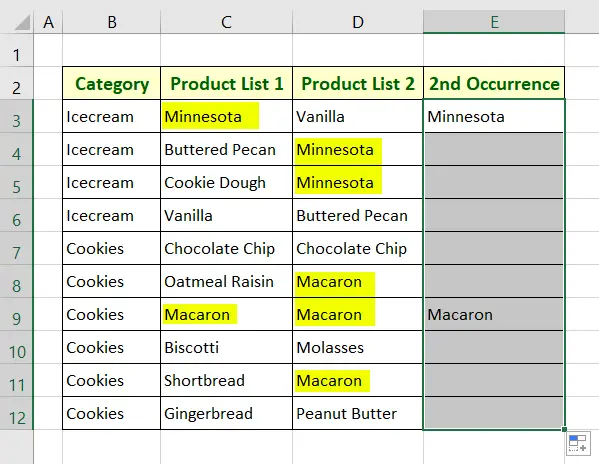### C. Return the Duplicates for the 3rd Occurrence

Use the following formula to return duplicates from Product List 1 having at least 3 copies existing in Product List 2.

Syntax

`=NthVlookup(lookup_value, table_array, col_index_num,occurrence)`

Usage Guide

Step_1: Insert the following formula in the top cell of column “3rd Occurrence”.

`=NthVlookup(C3,\$D\$3:\$D\$12,1,3)`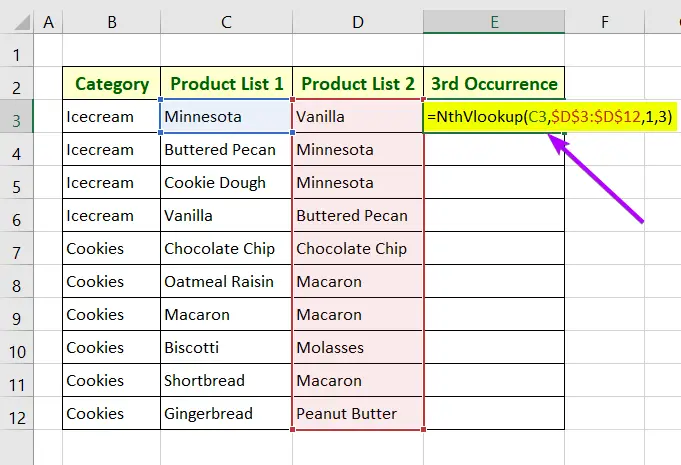Formula Breakdown

• C3 is the lookup value which is the top cell of the column Product List 1.
• \$D\$3:\$D\$12 is the table array which is the range of the column Product List 2.
• 1 is the column index number which refers to the first column in the range \$D\$3:\$D\$12.
• 3 is the occurrence number that refers to the 3rd occurrence.

Step_2: Press ENTER.

Step_3: Double-click on the Fill Handle icon to copy the formula down the entire column.

Final Result

In the 3rd Occurrence column, you will see only the duplicates from Product List 1 having at least 3 copies existing in Product List 2.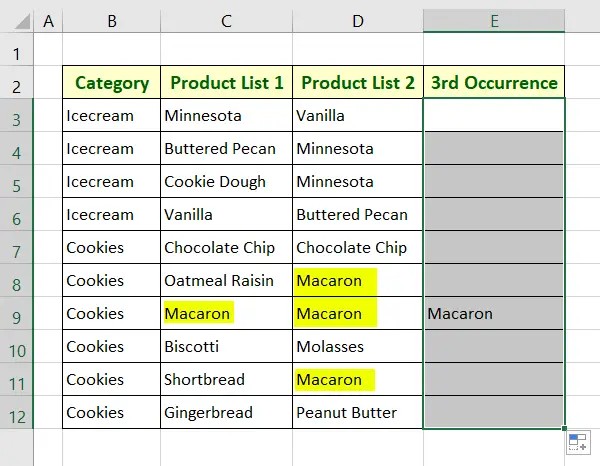🔗 5+ Formulas to Find Duplicates in One Column in Excel

## Conclusion

To sum up, I have discussed 8 scenarios to vlookup for duplicate values and return the matches in Excel. Leave a comment in the comment section below if you find any issues regarding the above cases.